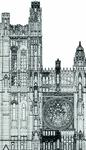proportion

(redirected from proportionability)
Also found in: Dictionary, Thesaurus, Medical, Legal.

proportion,

in mathematics, the equality of two ratiosratio.
The ratio of two quantities expressed in terms of the same unit is the fraction that has the first quantity as numerator and the second as denominator. For example, if in a group of 100 people 5 die, the ratio of deaths to the total number in the group is 5/100=1/20=.05.
. Two pairs of quantities a,b and c,d are in proportion if their ratios a/b and c/d are equal, i.e., if the equation a/b=c/d is true. For example, the lengths of two sides of any triangle and the lengths of the corresponding two sides of any similar (same-shaped) triangle are in proportion, for the ratio of the two sides of the first can be proved to be the same as the ratio of the two sides of the second. The proportion a/b=c/d was formerly written a:b::c:d and is read as "a is to b as c is to d." In this form it is customary to call b and c the means and a and d the extremes. These terms are used in the statement of the rule—the product of the means equals the product of the extremes. When the proportion is written in equation form, however, this rule is seen to be simply the result of a familiar algebraic operation. Similarly all the other rules stated for proportions become obvious when the proportion is written as an equation and the usual rules of algebra are applied. The special proportion a/b=b/(a+b) is known as the Divine Proportion, or Golden SectionGolden Section,
in mathematics, division of a line segment into two segments such that the ratio of the original segment to the larger division is equal to the ratio of the larger division to the smaller division.
.

Proportion

The ratio of one part to another, or its relationship to the whole; a comparison of parts as to size, length, width, and depth.Proportion

(1) In mathematics, a proportion is a statement of the equality of two ratios:The quantities a, b, c, and d are called the terms of the proportion; a and d are the extremes, and b and c are the means. It is a fundamental property of a proportion that the product of the means must equal the product of the extremes: bc = ad. This property is made use of in checking the correctness of a proportion and in expressing one of the terms by means of the others—for example,(2) In the plastic arts, proportion refers to the relation between the magnitudes of the elements of an artistic work or between the magnitudes of the individual elements and the work as a whole. A distinction is made between systems of proportion used in architecture and systems of proportion used in the depiction of the human body or face. The concept of proportion originated with the architects and artists of antiquity, who used certain modules and geometric constructions in creating their works. Besides systems of proportion based on ratios equal to integers or quotients of integers, systems involving irrational numbers were in wide use. An example is the golden section. Systems of proportion that reflected regularities actually existing in nature were often associated with mythological conceptions of the harmony of the universe. In modern architecture and design, an important problem is the development of systems of proportion under the conditions of the standardization of dimensions and parameters of products.

REFERENCES

Brunov, N. Proportsii antichnoi i srednevekovoi arkhitektury. [Moscow, 1936.]
Ghyka, M. Estetika proportsii v prirode i iskusstve. Moscow, 1936. (Translated from French.)
Mössel, E. Proportsii v anlichnosti i v srednie veka. Moscow, 1936. (Translated from German.)
Ocherki teorii arkhitekturnoi kompozitsii (collection). Moscow, 1960.
Mikhailov, B. P. Vitruvii i Ellada. Moscow, 1967.
Panofsky, E. “Die Entwicklung der Proportionslehre als Abbild der Stilentwicklung.” Monatshefte für Kunstwissenschaft, 1921, vol. 14, pp. 188–219.
Graf, H. Bibliographie zum Problem der Proportionen. Speyer, 1958.

proportion

[prə′pȯr·shən]
(mathematics)
The proportion of two quantities is their ratio.
The statement that two ratios are equal.

proportion

1. Maths a relationship that maintains a constant ratio between two variable quantities
2. Maths a relationship between four numbers or quantities in which the ratio of the first pair equals the ratio of the second pair
Site: Follow: Share:
Open / Close